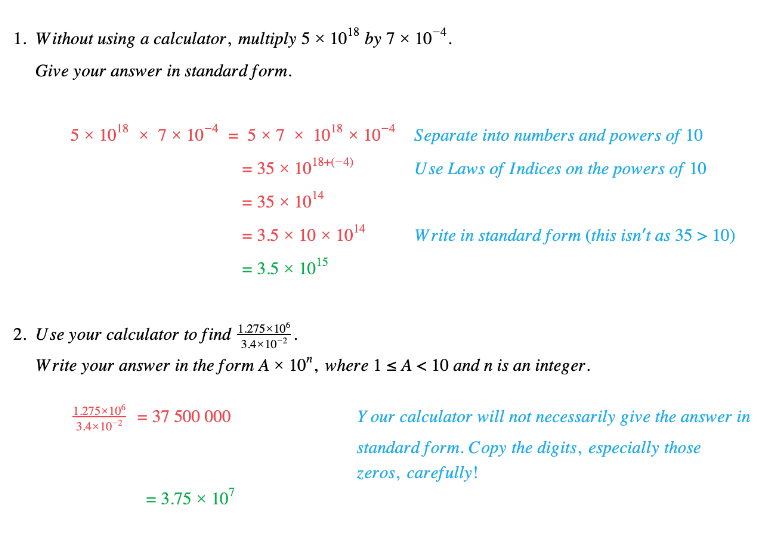# Edexcel IGCSE Maths复习笔记 1.13.1 Standard Form - Basics

Edexcel IGCSE Maths复习笔记 1.13.1 Standard Form - Basics

#### What is standard form?

• Standard Form (sometimes called Standard Index Form) is a way of writing very big and very small numbers using powers of 10

#### Why do we use standard form?

• Writing big (and small) numbers in Standard Form allows us to:
• write them more neatly
• compare them more easily
• and it makes things easier when doing calculations

#### How do we use standard form?

• Using Standard Form numbers are always written in the form:a × 10n
• The rules:
• 1 ≤ a < 10 so there is one non-zero digit before the decimal point
• n > 0 for LARGE numbers – how many times a is multiplied by 10
• n < 0 for SMALL numbers – how many times a is divided by 10
• Do calculations on a calculator (if allowed)

Otherwise follow normal rules (including indices) but adjust answer to fit Standard Form (move decimal point and change n

#### Worked Example